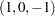Using the DESIGN Output Option

This example uses PROC TRANSREG and the DESIGN o-option to prepare an input data set with classification variables for the LOGISTIC procedure. The DESIGN o-option specifies that the goal is design matrix creation, not analysis. When you specify DESIGN, dependent variables are not required. The DEVIATIONS (or EFFECTS) t-option requests a deviations-from-meanscoding of the classification variables, which is the same coding the CATMOD procedure uses. PROC TRANSREG automatically creates a macro variable &_TrgInd that contains the list of independent variables created. This macro is used in the PROC LOGISTIC MODEL statement. (See Figure 93.75.) For comparison, the same analysis is also performed with PROC CATMOD. The following statements create Figure 93.75:

```title 'Using PROC TRANSREG to Create a Design Matrix';

data a;
do y = 1, 2;
do a = 1 to 4;
do b = 1 to 3;
w = ceil(uniform(1) * 10 + 10);
output;
end;
end;
end;
run;

proc transreg data=a design;
model class(a b / deviations);
id y w;
output out=coded;
run;

proc print;
title2 'PROC TRANSREG Output Data Set';
run;

title2 'PROC LOGISTIC with Classification Variables';

proc logistic;
freq w;
model y = &_trgind;
run;

title2 'PROC CATMOD Should Produce the Same Results';

proc catmod data=a;
model y = a b;
weight w;
run;
```

Figure 93.75 The PROC TRANSREG Design Matrix
 Using PROC TRANSREG to Create a Design Matrix PROC LOGISTIC with Classification Variables

The LOGISTIC Procedure

Model Information
Data Set WORK.CODED
Response Variable y
Number of Response Levels 2
Frequency Variable w
Model binary logit
Optimization Technique Fisher's scoring

 Number of Observations Read 24 24 375 375

Response Profile
Ordered
Value
y Total
Frequency
1 1 188
2 2 187

Probability modeled is y=1.

Model Convergence Status
Convergence criterion (GCONV=1E-8) satisfied.

Model Fit Statistics
Criterion Intercept
Only
Intercept
and
Covariates
AIC 521.858 524.378
SC 525.785 547.939
-2 Log L 519.858 512.378

Testing Global Null Hypothesis: BETA=0
Test Chi-Square DF Pr > ChiSq
Likelihood Ratio 7.4799 5 0.1873
Score 7.4312 5 0.1905
Wald 7.3356 5 0.1969

Analysis of Maximum Likelihood Estimates
Parameter DF Estimate Standard
Error
Wald
Chi-Square
Pr > ChiSq
Intercept 1 -0.00040 0.1044 0.0000 0.9969
a1 1 -0.0802 0.1791 0.2007 0.6542
a2 1 0.2001 0.1800 1.2363 0.2662
a3 1 -0.1350 0.1819 0.5514 0.4578
b1 1 -0.2392 0.1500 2.5436 0.1107
b2 1 0.3433 0.1474 5.4223 0.0199

Odds Ratio Estimates
Effect Point Estimate 95% Wald
Confidence Limits
a1 0.923 0.650 1.311
a2 1.222 0.858 1.738
a3 0.874 0.612 1.248
b1 0.787 0.587 1.056
b2 1.410 1.056 1.882

Association of Predicted Probabilities and
Observed Responses
Percent Concordant 54.0 Somers' D 0.163
Percent Discordant 37.8 Gamma 0.177
Percent Tied 8.2 Tau-a 0.082
Pairs 35156 c 0.581

 Using PROC TRANSREG to Create a Design Matrix PROC CATMOD Should Produce the Same Results

The CATMOD Procedure

Data Summary
Response y Response Levels 2
Weight Variable w Populations 12
Data Set A Total Frequency 375
Frequency Missing 0 Observations 24

Population Profiles
Sample a b Sample Size
1 1 1 31
2 1 2 31
3 1 3 34
4 2 1 26
5 2 2 33
6 2 3 37
7 3 1 36
8 3 2 29
9 3 3 28
10 4 1 26
11 4 2 35
12 4 3 29

Response Profiles
Response y
1 1
2 2

Maximum Likelihood Analysis
Maximum likelihood computations converged.

Maximum Likelihood Analysis of Variance
Source DF Chi-Square Pr > ChiSq
Intercept 1 0.00 0.9969
a 3 1.50 0.6823
b 2 5.64 0.0597
Likelihood Ratio 6 2.81 0.8329

Analysis of Maximum Likelihood Estimates
Parameter   Estimate Standard
Error
Chi-
Square
Pr > ChiSq
Intercept   -0.00040 0.1044 0.00 0.9969
a 1 -0.0802 0.1791 0.20 0.6542
2 0.2001 0.1800 1.24 0.2662
3 -0.1350 0.1819 0.55 0.4578
b 1 -0.2392 0.1500 2.54 0.1107
2 0.3434 0.1474 5.42 0.0199